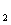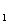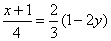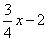ICSE Class 9 Graphical Solution New Pattern

## ICSE Class 9 Graphical Solution New Pattern By Clarify Knowledge

ICSE Class 9 Graphical Solution New Pattern 2022

## Chapter 27 - Graphical Solution (Solution of Simultaneous Linear Equations, Graphically) Exercise Ex. 27(A)

Question 1

1.Draw the graph for each equation, given

below:

(i) x = 5 (ii) x + 5 = 0

(iii) y = 7 (iv) y + 7 = 0

(v) 2x + 3y = 0 (vi) 3x + 2y = 6

(vii) x - 5y + 4 = 0 (viii) 5x + y + 5 = 0Solution 1

(i)

The graph x= 5 in the following figure is a straight line AB which is parallel to y axis at a distance of 5 units from it.

(ii)

x+5=0 Þx = -5

The graph x= -5 in the following figure is a straight line AB which is parallel to y axis at a distance of 5 units from it in the negative x direction.

(iii)

The graph y = 7 in the following figure is a straight line AB which is parallel to x axis at a distance of 7 units from it.

(iv)

y+7=0y = -7

The graph y = -7 in the following figure is a straight line AB which is parallel to x axis at a distance of 7 units from it in the negative y direction.

(v)

2x + 3y = 03y=-2x

(vi)

3x + 2y = 62y=6-3x

(vii)

x-5y+4=05y=4+x

(viii)

5x + y + 5 = 0y = -5x - 5

Question 2

Draw the graph for each equation given

below; hence find the co-ordinates of the

points where the graph drawn meets the co-

ordinate axes :

Solution 2

From the figure it is clear that, the graph meets the coordinate
axes at (3, 0) and (0, 5)

From the figure it is clear that, the graph meets the coordinate
axes at (-9, 0) and (0, 6)Question 3

Draw the graph of the straight line given by

the equation 4x - 3y + 36 = 0

Calculate the area of the triangle formed

by the line drawn and the co-ordinate axes.Solution 3

The straight line cuts the co-ordinate axis at A(0, 12) and B(-9, 0).

Question 4

Draw the graph of the equation

2x - 3y - 5 = 0

From the graph, find :

(i) x, the value of x, when y = 7

(ii) x, the value of x, when y = -5Solution 4

Question 5

Draw the graph of the equation

4x + 3y + 6 = 0

From the graph, find :

(i) y, the value of y, when x = 12

(ii) y, the value of y, when x = -6Solution 5

Question 6

Use the table given below to draw the graph.

From your graph, find the values of 'a' and

'b'.

State a linear relation between the variables

x and y.Solution 6

Question 7

Draw the graph obtained from the table

below:

Use the graph to find the values of a, b and

c. State a linear relation between the variables

x and y.Solution 7

Question 8

A straight line passes through the points

(2, 4) and (5, -2). Taking 1 cm = 1 unit;

mark these points on a graph paper and draw

the straight line through these points. If points

(m, -4) and (3, n) lie on the line drawn; find

the values of m and n.Solution 8

Question 9

Draw the graph (straight line) given by

equation x - 3y = 18. If the straight line

drawn passes through the points (m, -5) and

(6, n); find the values of m and n.Solution 9

Question 10

Use the graphical method to find the value

of k, if:

(i) (k, -3) lies on the straight line

2x + 3y = 1

(ii) (5, k - 2) lies on the straight line

x - 2y + 1 = 0Solution 10

(i)

(ii)

## Chapter 27 - Graphical Solution (Solution of Simultaneous Linear Equations, Graphically) Exercise Ex. 27(B)

Question 1

Solve, graphically, the following pairs of equations :

(i) x - 5 = 0                 (ii) 2x + y = 23

y + 4 = 0                         4x - y = 19

(iii) 3x + 7y = 27           (iv)Solution 1

(i)

(ii)

(iii)

(iv)

Question 2

Solve graphically the simultaneous equations

given below. Take the scale as 2 cm = 1 unit

on both the axes.

x - 2y - 4 = 0

2x + y = 3Solution 2

Question 3

Use graph paper for this question. Draw the

graph of 2x - y - 1 = 0 and 2x + y = 9 on

the same axes. Use 2 cm = 1 unit on both

axes and plot only 3 points per line.

Write down the co-ordinates of the point of

intersection of the two lines.Solution 3

Question 4

Use graph paper for this question. Take 2 cm = 2 units on x-axis and 2 cm = 1 unit on y-axis.

Solve graphically the following equations :

3x + 5y = 12; 3x - 5y + 18 = 0

(Plot only three points per line)Solution 4

Question 5

Use graph paper for this question. Take

2 cm = 1 unit on both the axes.

(i) Draw the graphs of x + y + 3 = 0 and

3x - 2y + 4 = O. Plot only three points per line.

(ii) Write down the co-ordinates of the point

of intersection of the lines.

(iii) Measure and record the distance of the

point of intersection of the lines from the

origin in cm.Solution 5

(i)

(ii)

(iii)

Question 6

The sides of a triangle are given by the

equations y - 2 = 0; y + 1 = 3 (x - 2) and

x + 2y = O.

Find, graphically :

(i) the area of triangle;

(ii) the co-ordinates of the vertices of the

triangle.Solution 6

(ii)

Question 7

By drawing a graph for each of the equations

3x + y + 5 = 0; 3y - x = 5 and 2x + 5y = 1

on the same graph paper; show that the lines

given by these equations are concurrent (i.e.

they pass through the same point).

Take 2 cm = 1 unit on both the axes.Solution 7

Question 8

Using a scale of 1 cm to 1 unit for both the

axes, draw the graphs of the following

equations: 6y = 5x + 10, y = 5x - 15.

From the graph find :

(i) the co-ordinates of the point where the

two lines intersect;

(ii) the area of the triangle between the lines

and the x-axis.Solution 8

(i)

(ii)

Question 9

The cost of manufacturing x articles is

Rs.(50 + 3x). The selling price of x articles is

Rs.4x.

On a graph sheet, with the same axes, and

taking suitable scales draw two graphs, first

for the cost of manufacturing against no. of

articles and the second for the selling price

against number of articles.

(i) No. of articles to be manufactured and

sold to break even (no profit and no loss),

(ii) The profit or loss made when (a) 30

(b) 60 articles are manufactured and soldSolution 9

(i)

No. of articles to be manufactured and sold are 50 when there is no loss and no profit.

C.P = S.P = Rs.200

(ii)

(a)

On article 30,

C.P = Rs.140 and S.P. = 120

Therefore Loss = 140 - 120 = Rs.20

(b)

On article 60,

C.P.=Rs.230 and S.P.= Rs.240

Therefore Profit = 240 - 230 = Rs.10Question 10

Find graphically, the vertices of the triangle

whose sides have the equations 2y - x = 8;

5y - x = 14 and y - 2x = 1 respectively.

Take 1 cm = 1 unit on both the axes.Solution 10

Question 11

Using the same axes of co-ordinates and the

same unit, solve graphically :

x + y = 0 and 3x - 2y = 10.

(Take at least 3 points for each line drawn).Solution 11

Question 12

Solve graphically, the following equations.

x + 2y = 4; 3x - 2y = 4.

Take 2 cm = 1 unit on each axis.

Also, find the area of the triangle formed by

the lines and the x-axis.Solution 12

Therefore the solution of the given system of equations is (2,1).

Question 13

Use the graphical method to find the value of

'x' for which the expressionsandare equal.Solution 13

Question 14

The course of an enemy submarine, as plotted

on rectangular co-ordinate axes, gives the

equation 2x + 3y = 4. On the same axes, a

destroyer's course is indicated by the graph

x - y = 7. Use the graphical method to find

the point at which the paths of the submarine

and the destroyer intersect ?Solution 14

error: Content is protected !!